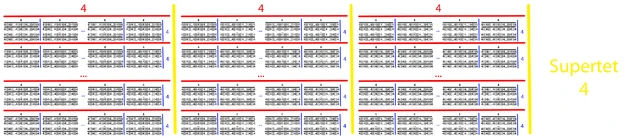11,053 Pages

Supertet is equal to {4,4,4,4}, {4,4(1)2} or {4,2/2} in BEAF.. It can also be written 4{{{{4}}}}4, or 4 & 4, using the array of operator. The term was coined by Jonathan Bowers. It is decently close to a well-known combinatorial constant, Harvey Friedman's n(4).

Supertet can be computed in 3-bracket operator notation using the following process:

• $$t_1 = 4$$
• $$t_2 = 4\{\{\{4\{\{\{4\{\{\{4\}\}\}4\}\}\}4\}\}\}4$$
• $$t_3 = 4\{\{\{4\{\{\{4\cdots\{\{\{4\}\}\}4\}\}\}\cdots\}\}\}4$$ with $$t_2$$ 4's from the center out.
• $$t_4 = 4\{\{\{4\{\{\{4\cdots\{\{\{4\}\}\}4\}\}\}\cdots\}\}\}4$$ with $$t_3$$ 4's from the center out.
• etc.
• Supertet is $$t_{t_{t_{\cdots1}}}$$, where there are $$t_{t_{t_{\cdots1}}}$$ $$t$$s, where there are $$t_{t_{t_{t_1}}}$$ $$t$$s.

Supertet is comparable to $$f_{\omega^2}(4)$$ in the fast-growing hierarchy.Visual representation of a supertet using extended operator notation

## Etymology

The name of this number is based on the words "super" and "tetra-" (four).

## Approximations

Notation Approximation
Hyper-E notation E4##4##4##4##5
Chained arrow notation $$4\rightarrow 4\rightarrow 4\rightarrow 4\rightarrow 4\rightarrow 5$$
Strong array notation s(4,4,5,4)
Fast-growing hierarchy $$f_{\omega^2}(4)$$
Hardy hierarchy $$H_{\omega^{\omega^2}}(4)$$
Slow-growing hierarchy $$g_{\varphi(3,\omega,0)}(4)$$

## Sources

1. Bowers, JonathanInfinity Scrapers. Retrieved January 2013.

## See also

Tritri series: tritri · tritet · tripent · trisept · tridecal · grand tridecal
Tetratri series: tetratri · supertet · general(plex)
Pentatri series: pentatri · superpent · pentadecal(plex)
Hexatri series: hexatri · superhex · hexadecal(plex)
Heptatri series: heptatri · supersept · heptadecal
Iteral series: superoct · octadecal · superenn · ennadecal · iteral · ultatri
Dupertri series: dupertri · duperdecal · truperdecal · quadruperdecal
Latri series: latri · emperal(plex) · hyperal(plex) · admiral
Dutritri series: dutritri · dutridecal
Dimentri series: dimentri · dulatri · trilatri · trimentri
Triakulus series: triakulus · tridecatrix
Big boowa series: big boowa · great big boowa · grand boowa
Tiaokhiao's extensions: trihex · trioct · triennet · triundecal · tridodecal · tritriplex · tritriplexian · grand tritri · tetrapent · tetrahex · grand tetratri · octatri · enneatri · decatri · undecatri · dodecatri

Community content is available under CC-BY-SA unless otherwise noted.